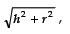Courses

# Facts That Matter- Surface Areas and Volumes Class 10 Notes | EduRev

## Class 10 : Facts That Matter- Surface Areas and Volumes Class 10 Notes | EduRev

The document Facts That Matter- Surface Areas and Volumes Class 10 Notes | EduRev is a part of the Class 10 Course Mathematics (Maths) Class 10.
All you need of Class 10 at this link: Class 10

Facts that Matter
An object having definite shape and size is called a solid.
Solids like a book, a tile, a match box, an almirah, a room, etc. are called cuboids.
Solids like dice, ice-cubes, sugar-cubes, etc. are called cubes.
Solids like jars, circular pillars, circular pipes, circular pencils, gas jars, road rollers, etc. are called cylinders.
Solids like conical tents, ice-cream cones, funnels, etc. are called cones.
Solids like cricket balls, footballs etc., are called spheres.
When a cone is cut by a plane parallel to the base of the cone then the portion between the plane and the base is called the frustum of the cone

NOTE:
I. Solids like rubber tubes, iron pipes etc., are called hollow cylinders.
II. A plane through the centre of a sphere cuts it into two equal parts. Each part is called a hemisphere.
We also know that:
I. For a cuboid of length = l, breadth = b and height = h, we have:
(i) Volume = (l × b × h) cu. units.
(ii) Lateral surface area = [2 (l + b) × h] sq. units.
(iii) Total surface area = [2 (lb + bh + hl] sq. units.

II. For a cylinder of base radius = r and height (or length) = h, we have:
(i) Volume = πr2h cu. units.
(ii) Curved surface area = 2πrh sq. units.
(iii) Total surface area = 2πr (h + r) sq. units.

III. For a hollow cylinder having external radius = R, internal radius = r and height = h, we have:
(i) Volume
= [External volume] − [Internal volume]
=(πR2h − πr2h) cu. units = π(R2 − r2) cu. units.
(ii) Curved surface area
= [External S.A.] + [Internal S.A.]
=[2πRh + 2πrh] sq. units = 2πh (R + r) sq. units
(iii) Total surface area
= (curved S.A.) + (area of the base-ring)
= [(2πRh + 2πrh) + 2 (πR2 − πr2)] sq. units
= [(2πh (R + r) + 2π (R2 − r2)] sq. units

IV. For a cone of base radius = r, height = h and slant height l =we have:
(i) Volume = 1/3 πr2h cu. units.
(ii) Curved surface area = πrl sq. units.
(iii) Total surface area = [Curved surface area] + [Area of base]
= [πrl + πr2] sq. units = πr (l + r) sq. units.

V. For a sphere of radius r, we have:
(i) Volume = 4/3 πr3 cu. units.

VI. For a hemisphere of radius r, we have:
(i) Volume = 2/3 πr3 cu. units.
(ii) Surface area = 2πr2 sq. units.
(iii) Total surface area = 3πr2 sq. units.

VII. For a frustum of a cone of base radius = R, top radius = r, height = h and slant height = l, we have:
(i) Volume  = πh/3 [R2 + r2 + Rr] cu. units.
(ii) Lateral surface area
= πl (R + r) sq. units, where l2 = h2 + (R − r)2
(iii) Total surface area
= [(area of base) + (area of top) + (lateral surface area)]
= [πR2 + πr2 + πl (R + r)] sq. units.
= π [R2 + r2 + l (R + r)] sq. units.

Offer running on EduRev: Apply code STAYHOME200 to get INR 200 off on our premium plan EduRev Infinity!

## Mathematics (Maths) Class 10

178 videos|268 docs|103 tests

,

,

,

,

,

,

,

,

,

,

,

,

,

,

,

,

,

,

,

,

,

;